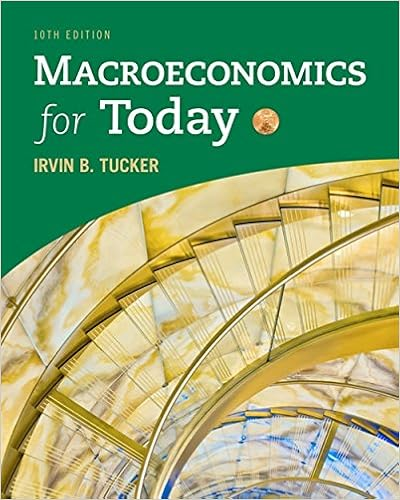# Given that the mpc8 we know that 2 of every dollar

• Homework Help
• 20
• 96% (124) 119 out of 124 people found this document helpful

This preview shows page 3 - 7 out of 20 pages.

##### We have textbook solutions for you!
The document you are viewing contains questions related to this textbook.The document you are viewing contains questions related to this textbook.
Chapter 8 / Exercise 13
Macroeconomics for Today
TuckerExpert Verified
5.Given that the MPC=.8, we know that .2 of every dollar increase in gross income is saved. Since the increase in income is \$100, we know the leakage due to savings is:
##### We have textbook solutions for you!
The document you are viewing contains questions related to this textbook.The document you are viewing contains questions related to this textbook.
Chapter 8 / Exercise 13
Macroeconomics for Today
TuckerExpert Verified
goods. Multiplying by 100 we have the following: Y up by 100, savings up by 20, taxes up by 25, imports up by 20, consumption up by 35Points Earned:3.0/3.0Correct Answer(s):C6.To find out how much consumption increases we need to take the increase in income (\$100) and subtract out the leakages. So take the \$100 and subtract your answers from #3, #4 and#5 above. When income increases by \$100, consumption increases by:
Points Earned:3.0/3.0
7.What would happen to the multiplier if the mpi rises to .25? Round to 2 decimal places.
Points Earned:3.0/3.0Correct Answer(s):8.What would happen to the size of the leakage if the mpi rises to .25?AA) this would reduce the size of the leakageB) this would increase the size of the leakage Feedback: This would increase the size of the leakage - if you do the math, the new multiplier is 1.54.Correct Answer(s):B9.In this question, we are going dig deeper into the Taylor Rule and it variants (modifications).Federal Reserve data from October 1, 2011:Potential GDP growth y* = 1.7%Actual GDP Growth yA= 2.0% Inflation PCE (actual inflation) πA= 2.6% Effective Federal funds Rate = .07%As Taylor assumed, we assume the equilibrium real rate of interest r* = 2% and the optimal inflation rate, the target inflation rate π* is also equal to 2%.The standard (original) Taylor rule formula: ifTR= r* + πA+ 0.5[πA- π*] + 0.5 [ yA- y*]
Points Earned:3.0/3.0
Using the 'standard' Taylor rule from above and using the data provided, what is the federal funds rate implied by the 'standard' Taylor Rule?
Feedback:Data as of 4th quarter 2011Original Taylor Rule: iffTR= r* + πA+ 0.5[πA- π*] + 0.5 [ yA- y*]5.05 = 2 + 2.6 + .5[2.6 - 2] + .5[2.0 - 1.7]Points Earned:4.0/4.0Correct Answer(s):D
•••